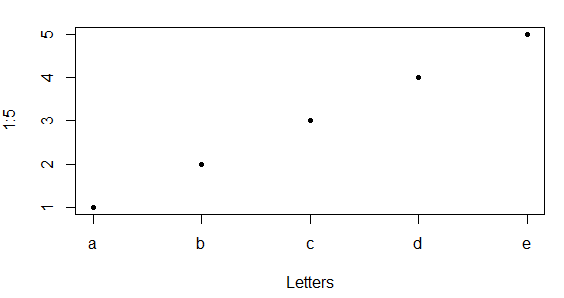2 views

I have a question regarding the command plot().

Is there a way to fully eliminate the x-axis and replace it with own values? I know that I can get rid of the axis by doing

plot(x,y, xaxt = 'n')

and then add an axis with

axis(side = 1 etc.)

However, when I add the axis, obviously it still refers to the data plotted as 'x'. I would only like to plot the 'y'-values and add the x-axis my own in the sense of just "drawing" the x-axis with own values specified. Is there any way to do that?

The background of this question is that my two data frames differ in their length and therefore I cannot plot them.

by

To replace x-axis values with your own values, you can use the axis function as follows:

plot(1:5, xaxt = "n", xlab='Letters', pch = 20)

axis(1, at=1:5, labels=letters[1:5])

Output: